## Sunday, December 18, 2005

### Continued Fractions and Matrices

Matrices are very useful when analyzing continued fractions. In previous blogs, I wrote about the continued fractions approximation function and the continued fractions basic properties. Today, I will show how matrices can be used to reason with continued fractions.

Today's blog is based on H. M. Stark's An Introduction to Number Theory. If you are not familiar with Continued Fractions, start here. If you are not familiar with the Continued Fraction approximation algorithm, start here.

A continued fraction breaks a real number into a series of integers such as a0, a1, ... an. It makes sense that we can use matrices to reason about them.

In today's blog, I will focus on the simplest properties of matrices. For example, I will only deal with two types of matrices:

1 x 2 matrices such as (5,6)

2 x 2 matrices

For those who would need a review of simple matrices, matrix products, determinants, inverses, or some basic lemmas, start here.

To represent, continued fractions as matrices, we define the following:

(a) An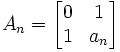NOTE: an is taken directly from the continued fraction. See here for details on how this is generated.

(b) Mn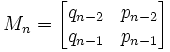NOTE: pn, qn are generated based on the continued fraction approximation function. See here for details on how these two values are generated.

(c) γn

γn = qn-2 + αnqn-1

NOTE: αn is generated based on the continued fraction for α (see here). qn is generated based on the continued fraction approximation algorithm (see here).

Using these definitions, I will go through some lemmas that will be useful in solving Pell's equation.

Lemma 1: Mn+1 = AnMn

(1) AnMn =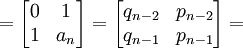NOTE: By the definitions above.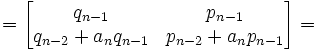NOTE: By carrying out the matrix product [see here for review if needed]NOTE: By applying the definitions of pn and qn [see here for details]

= Mn+1 [By the definition above]

QED

Lemma 2: γn(1,α) = (1,αn)Mn

(1) (1,αn)Mn =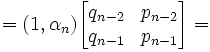= (qn-2 + αnqn-1 , pn-2 + αnpn-1) [See here to review matrix products]

= (qn-2 + αnqn-1)(1,α) [Since α * (qn-2 + αnqn-1) = pn-2 + αnpn-1; see here]

= γn(1,α)

QED

Lemma 3: α is irrational → γn ≠ 0

(1) Since α is irrational, αn is irrational. (See here for proof; only rational numbers give way to rational αn)

(2) Assume γn = 0.

(3) Then, qn-2 + αnqn-1 = 0.

(4) Then qn-2=0 and qn-1 = 0. [See here for proof]

(5) q-1 = q1 - q0a1 = a1 - a1(1) = 0
q-2 = q0 - q-1a0 = 1 - 0*a0 = 1

NOTE: See here for review of qn

(6) We also know for all values n ≥ 1, that qn ≥ 1 (see here).

(7) So (#4) is impossible and we reject our assumption.

QED

Lemma 4: det(Mn) = (-1)n

(1) det(Mn) = pn-1qn-2 - pn-2qn-1 (See here for a review of determinants)

(2) So, det(Mn) = (-1)n by a previous result. (See here)

QED

Lemma 5: Mn+1 = AnAn-1...A1A0

(1) Mn+1 = AnMn (From Lemma 1 above)

(2) Applying Lemma 1 again gives us:

Mn+1 = AnAn-1Mn-1

(3) Since n is an integer greater than 0, we can keep on doing this until eventually we get:

Mn+1 = AnAn-1...A1A0

NOTE:

(a) p-1 = p1 - p0a1 = a0a1 + 1 - a0a1 = 1.

(b) q-1 = 0 (see Lemma 3 above)

(c)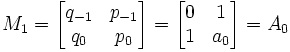QED

Lemma 6: Mn-1 is a 2 x 2 matrix of integers.

Mn-1 =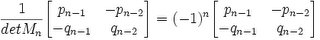NOTE: det(Mn) = (-1)n from Lemma 4 above.

QEDAnonymous said...

This site is terrific. Thanks for putting in all the effort.

There are some minor typos on this page. Near the top is a line that reads:

1 x 2 matrics such as (5,6)

The word "matrics" is misspelled. Also, the first 2x2 matrix under Lemma 1 has p_n-2 in the lower right entry, when it should be p_n-1.

Larry Freeman said...

Hi Bob,

Thanks very much for your feedback on the site! :-)

I also appreciate you pointing out the typos. I just fixed them.

Cheers,

-Larry

Scouse Rob said...

In the first image of Lemma 1:

There is an equals sign between the two matrices instead of a multiplication sign.

Rob